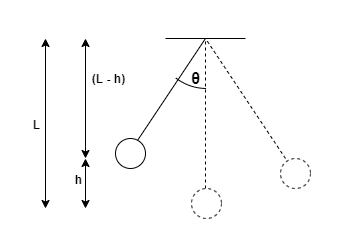# Problem: A pendulum is made by tying a 420g ball to a 51 cm long string. The pendulum is pulled 25 degrees to one side, then released.(a) what is the ball's speed at the lowest point of its trajectory?(b) To what angle does the pendulum swing on the other side?

###### FREE Expert Solution

Kinetic energy:

$\overline{){\mathbf{K}}{\mathbf{E}}{\mathbf{=}}\frac{\mathbf{1}}{\mathbf{2}}{\mathbf{m}}{{\mathbf{v}}}^{{\mathbf{2}}}}$

Gravitational potential energy:

$\overline{){\mathbf{U}}{\mathbf{=}}{\mathbf{m}}{\mathbf{g}}{\mathbf{h}}}$(a)

First, we need to determine the potential energy of the pendulum at 25°. However, the height, h, is unknown.

89% (258 ratings)###### Problem Details
A pendulum is made by tying a 420g ball to a 51 cm long string. The pendulum is pulled 25 degrees to one side, then released.
(a) what is the ball's speed at the lowest point of its trajectory?
(b) To what angle does the pendulum swing on the other side?